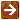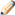﻿ Accrual Calculations

#### Accrual CalculationsUnless otherwise indicated, all calculations adhere to the standard rounding rules. For information on those rules, see Standard Rounding Rules.

The information required for calculating and recording accruals is stored in three tables.

The Security table contains information for each security regarding the frequency of payments, next payment date, the ex-dividend date, and dividend amount or annual payment rate.

Each security has a class code defined by a Class Code record that contains information about the type of accrual, if any, to calculate for the security.

Actual accrual amounts are calculated for individual holdings and stored in the Holding Tax Lot table.

Calculations vary according to the accrual type. However, for all accrual types, when the divisor is the number of days in the year, the system uses 365 except for a leap year, in which case the system uses 366. Additionally, all calculations are done by security and reporting of totals processed is by security.

Automated cash management calculations are performed on securities having class codes with an accrual method of 'A' (Automated Cash Management). The calculations are as follows:

For each Holding Tax Lot record for a security for which the number of holding units is greater than zero:

Accrual Amount = (Holding Units x Rate) ÷ Number of Days in Calendar Year

In the Holding Tax Lot record, add the calculated accrual amount to the accrued income due in the Holding Lot record.

Dividend calculations are performed as follows on securities having class codes with an accrual method of 'D' (Dividend) on the ex-dividend date:

For each Holding Tax Lot record for a security for which the number of holding units is greater than zero:

Accrual Amount = Holding Units x Security Dividend Amount

In the Holding Tax Lot record, add the calculated accrual amount to the accrued income due in the Holding Lot record.Reminder: Dividends accrue periodically as described above. They do not accrue daily.

Treasury notes and bonds calculations are performed on securities having class codes with an accrual method of 'T' (Treasury Notes and Bonds). The calculations are as follows:

Number of Days in Calculation Interval = Number of days between last date income was paid and the next pay date, based on the frequency code for the security. For example, if the payments are March 15th and September 15th, one six month interval will be March 16th to September 15th (184 days) and the other interval will be September 16th to March 15th (181 days or 182 in a leap year).

For each Holding Tax Lot record for a security where the number of holding units are greater than zero:

Accrual Amount = (Holding Units x [Security Rate ÷ 2]) ÷ Number of Days in Calculation Interval

In the Holding Tax Lot record, add the calculated accrual amount to the accrued income due in the Holding Lot record.

Time deposit calculations are performed on securities having class codes with an accrual method of 'M' (Market). The calculations are as follows:

For each Holding Tax Lot record for a security where the number of holding units are greater than zero:

Accrual Amount = (Holding Units x Rate) ÷ Number of Days in Calendar Year

In the Holding Tax Lot record, add the calculated accrual amount to the accrued income due in the Holding Lot record.

More: